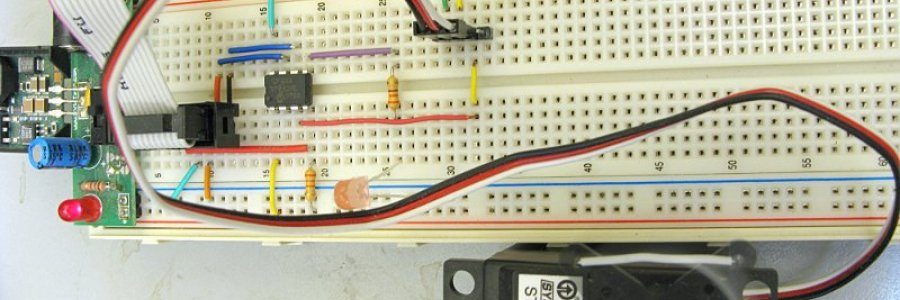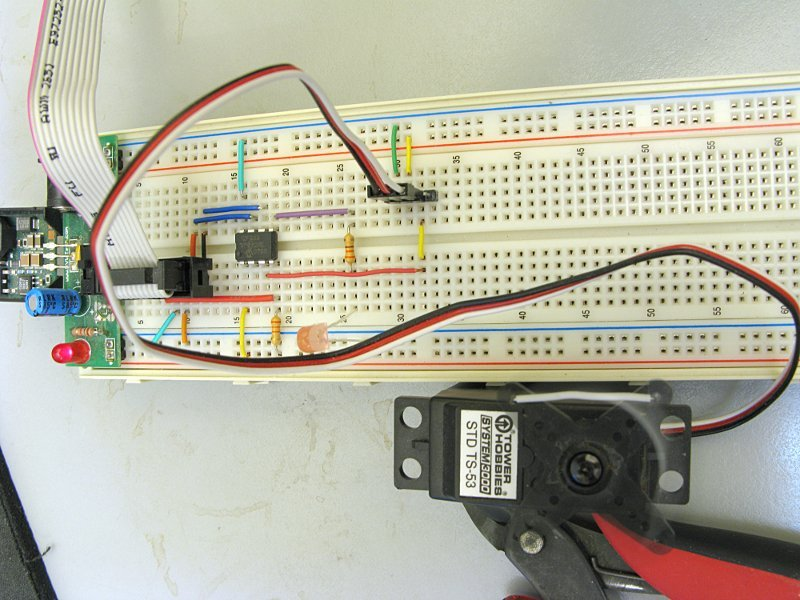#### Servo Play With the PIC 12F683A guy emailed me wanting to know how to write C code to do a servo slowdown device like this PICAXE one by Kevin Goom. So I started small and worked up to a full port of the PICAXE code in C. The only thing I left out is the indicator LED, but that's simple enough to add later.

I started out by just using the 12F683 to control a servo on GP4.This code uses Timer1 in compare mode with special event trigger enabled. Special event trigger resets Timer1 and generates a CCP1 interrupt when the Timer1 counter value matches the value in CCPR1. Each time the interrupt is triggered it toggles the servo pin and sets CCPR1 to the next required value to generate a proper 20ms period servo pulse-train.

``````#include <system.h>

#pragma DATA _CONFIG, _INTOSCIO &_MCLRE_ON & _WDT_OFF
#pragma CLOCK_FREQ 8000000

volatile int servo;         //servo position

void main()
{
int i;
osccon = 0b01110001;    //set int osc to 8MHz
cmcon0 = 7;             //disable comparator
ansel = 0;              //disable A/D - digital I/O
trisio = 0b00001000;    //set data directions
ccp1con = 0b00001011;   //special event trigger
t1con = 0b00010001;     //Timer1 enable, pre=2
pie1.CCP1IE = 1;        //enable ccp1 interrupt
intcon.PEIE = 1;        //enable peripheral interrupts
intcon.GIE = 1;         //enable global interrupts

servo = 260;
while(1){
delay_s(2);
for(i=260;i<2150;i+=10){
servo = i;
delay_ms(35);
}
delay_s(1);
servo = 260;
}
}

void interrupt(void)
{
int i;
if(gpio.GP4){
gpio.GP4 = 0;
i = 20000 - servo;
LOBYTE(ccpr1l,i);
HIBYTE(ccpr1h,i);
}
else{
gpio.GP4 = 1;
LOBYTE(ccpr1l,servo);
HIBYTE(ccpr1h,servo);
}
pir1.CCP1IF = 0;        //clear interrupt flag
}``````
##### The code in action:

That was pretty easy, so I decided to rewrite to control the servo with Timer2, which is 8-bit (Timer1 is 16-bit). This took a bit more work in the ISR, but is necessary as I will be needing Timer1 for capture duties later. (Turns out I didn't end up using Timer2 in the final program, but learning how to control a servo with an 8-bit timer was entertaining.)

``````#include <system.h>

#pragma DATA _CONFIG, _INTOSCIO &_MCLRE_ON & _WDT_OFF
#pragma CLOCK_FREQ 8000000

bit longflag = 0;
unsigned char count = 0;
volatile unsigned char servo;

void main()
{
int i,j;
osccon = 0b01110001;    //set int osc to 8MHz
cmcon0 = 7;             //disable comparator
ansel = 0;              //disable A/D - digital I/O
trisio = 0b00001000;    //set data directions
t2con = 0b00000110;     //timer2 enable, pre 16
pr2 = 187;              //timer2 period = 1.5ms
pie1.TMR2IE = 1;        //enable timer2 match interrupt
intcon.PEIE = 1;        //enable peripheral interrupts
intcon.GIE = 1;         //enable global interrupts

while(1){
servo = 50;
delay_s(1);
servo = 250;
delay_s(1);
}
}

void interrupt(void)
{
unsigned char i;
if((gpio.GP4 == 1) && (longflag == 0)){
gpio.GP4 = 0;
longflag = 1;
count = 9;
pr2 = 250;
}
else if(longflag){
count -= 1;
if(count == 0){
pr2 = 255 - servo;
longflag = 0;
}
}
else{
gpio.GP4 = 1;
pr2 = servo;
}
pir1.TMR2IF = 0;
}``````